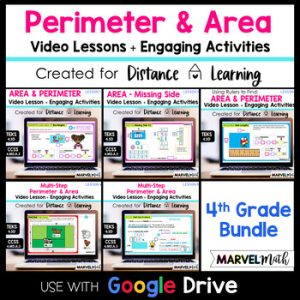Save 10% on your first order with code EXTRA10

# Area and Perimeter of Rectangles – Digital Activities + Video Lesson

\$4.00\$14.00
SKU: 5423310 Categories: ,

## Description

The fun and engaging Area and Perimeter of Rectangles Practice Activities and Video Lesson are Perfect for Distance Learning. Students will learn how to calculate the Perimeter and Area of Rectangles using formulas. These Simple and Easy-to-Use Google Slides are designed for 4th grade students and align to these standards: TEKS 4.5D and CCSS 4.MD.A.3.

This is Lesson 1 in a 4th Grade Perimeter/Area Series.

Here are links to the other lessons:

⭐ Student Devices must have access to You-Tube to view the Video Lesson ⭐

The Video Lesson:

• Teaches students how to use formulas to find the perimeter and area of rectangles and squares
• Uses a graphic organizer to help students sort and visualize information
• Walks students through the Google Slides activities they will complete
• Demonstrates how to use the Shape Tool in Google Slides
• Is approximately 13 minutes

⭐ Many of the slides are self-checking, so students can work through the activity with confidence.

Topics and Activities Include:

• Creating Multiplication Equations to Find Area
• Creating Addition Equations to Find Perimeter of Rectangles
• Creating Multiplication Equations to Find Area and Perimeter of Squares
• Fun Google Slides Activities with movable pieces
• Application of Learning in Real-World Word Problems
• Word Problems have optional Step-by-Step support

What’s Included?

• Video Lesson with Activity Instructions
• 11 Interactive Activities
• Teacher Key in separate Google Slides™ doc that can be assigned to students to check their work at home with their parents